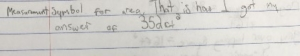Congratulations to the Kindergarten winner of the Problem of the Month competition for January! The winner is Dylan from Mrs. Mohammed’s classroom at Turner/Bartels K-8.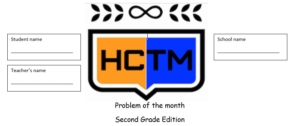Congratulations to the 2nd grade winner of the Problem of the Month competition for January! The winner is Abhijay from Mrs. Park’s classroom at Turner/Bartels K-8 School.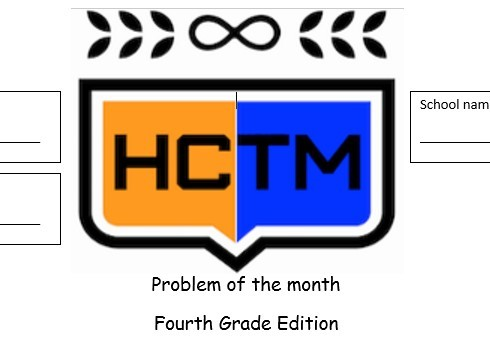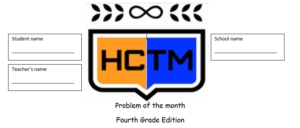Congratulations to the 4th Grade winner of the Problem of the Month competition for January! The winner is Sebastian from Mrs. King’s classroom at Claywell Elementary.Stenhouse Publishing is offering a free preview of the new book, Beyond Answers by Mike Flynn.

About the Book: The Standards for Mathematical Practice are written in clear, concise language. Even so, to interpret them and visualize what they mean for your teaching practice isn’t always easy. In this practical, easy-to-read book, Mike Flynn provides teachers with a clear and deep sense of these standards and shares ideas on how best to implement them in K–2 classrooms.

Take advantage of checking this preview out while it lasts by clicking here.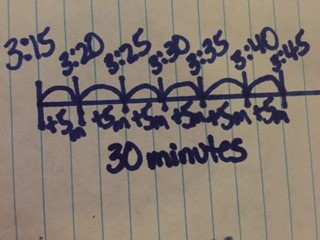To solve story problems involving elapsed time, encourage students to draw open number lines to help students conceptually understand the concept. This could prevent common misconceptions students may have when determining elapsed time.

Make sure to include different types of problems that require students to determine the start time, end time or elapsed time. See the examples of the different types of questions below:

Determine the Elapsed Time:

Riley went to the playground at 3:15. He left the playground at 3:45. How long did Riley spend at the playground?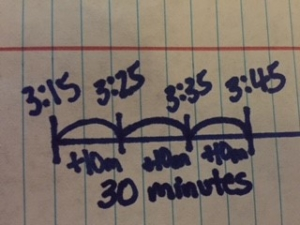Determine the End Time:

Riley went to the playground at 3:15. He spent 30 minutes at the playground. What time did Riley leave the playground?Determine the Start Time:

Riley spent 30 minutes at the playground. He left the playground at 3:45. What time did Riley get to the playground?It’s helpful to give students similar problems with the same topic, like the ones shown above, to have students sort and determine if they are finding the elapsed time, start time or end time. Encourage students to prove their answer with a number line.

It’s also important to share and discuss different strategies for solving for the elapsed, start or end times. For example, in the problem “Riley went to the playground at 3:15. He left the playground at 3:45. How long did Riley spend at the playground?”

One student below solved it with increments of 10, where another student solved it with increments of 5. Discuss which is most efficient and by showing more than one way, it could help other students have access to the more efficient strategy.Here are some example elapsed time problems from item specifications where students are asked to: determine the end time, determine elapsed time, use a number line, and use a table: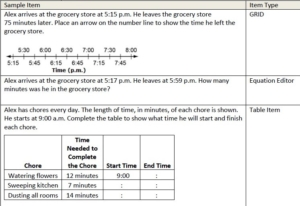For more information on using a number line to determine elapsed time, visit https://learnzillion.com/lesson_plans/9023-solving-elapsed-time-word-problems-to-the-nearest-minute.

For more lesson ideas on using a number line to determine elapsed time, visit NCTM Illuminations at http://illuminations.nctm.org/Lesson.aspx?id=3966.Telling a time is an important concept for everyday life. It’s also one that students some time struggle with if they don’t understand how a clock works.

In 3rd grade, students are expected to:
• Tell and write time to the nearest minute
• Use analog and digital clocks to tell time
• Measure time intervals in minutes
• Solve word problems that involve addition and subtraction of time intervals in minutes and represent the problems on a number line.

Using a linear number line model can sometimes help with misconceptions students have about telling time. Here are some ideas on how you can relate a number line and a clock to help students tell time:

Explore Time in a Number line format to encourage skip counting.
1. Create a number line with the intervals for the hours on a clock.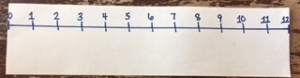2. Create a number line with the intervals for the minutes on a clock.3. Compare the two number lines and encourage students to look for patterns.4. Pose questions for students to compare how hours and minutes relate and work on a clock, such as:
o How many minutes relate to the 3rd hour?
o How many hours relate to 40 minutes?
o What pattern do you notice on the minute hand number line?
o What pattern do you notice on the hour hand number line?

5. Extend to show an hour hand on the hour number line and a minute hand on the minute number line. Have the students determine the time and record it digitally.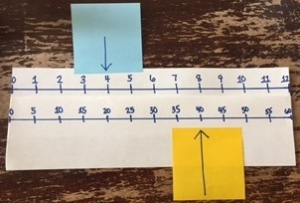6. This could also be done by making the linear clock into a circular clock. Use index cards to record each hour. Then attach the times with string to change it to a circular clock. Then explore time by just using the hour hand to determine time to the nearest and time to the nearest half hour. Could also include the minute and hour hand to use patterns to determine the time.For more information on relating a Number Line to a Clock, visit https://learnzillion.com/resources/64146-relating-skip-counting-to-time.We are all familiar with the term “reading first” and you can’t argue that literacy is important. However, when it comes to kids making more money later in life, more math may mean more money.

A recent study published by a Harvard professor seems to conclude that the more math classes a student takes, the more likely their income is to rise after graduation. This would also correspond to research done in the state of California in the early 2000’s. After controlling for other factors, just one extra course in algebra or geometry can be associated with 6.3% higher earnings!

What does this mean for us as elementary math teachers? First of all, we want to be sure we are providing all students with the benchmarks needed in mathematics in grades K-5 so that they have the foundation needed for high math classes in middle school, high school, and college (should they choose). Secondly, we must ensure that students learn how to be great thinkers and problems solvers now, so they can more easily make the transition to higher level classes in the future. Finally, when kids ask why is math important, just ask them if they think making money is important!
Unless you want to be a math teacher, it probably is! 😉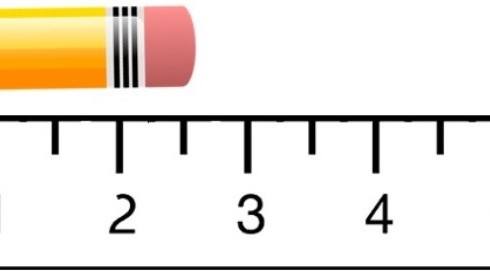Students in 3rd grade are expected to measure length to the nearest whole inch, half inch and quarter inch.

In order for students to be successful in measuring length and representing that length as fractions, consider using paper rulers to explore and compare the different fractional unit lengths using the steps below.

1. Give each student 3 different paper rulers.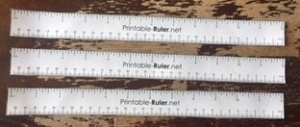2. Have the students highlight the whole units on one ruler, half units on another ruler, and the quarter units on the last ruler.3. Give various objects for students to measure to the nearest whole inch, nearest half inch, and nearest quarter inch and record in a chart.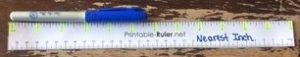4. From there, have students compare and contrast which units are the most precise length and how the measurements compare when you’re focusing on a specific fraction of an inch.

5. Spend time discussing how the length of the object does not change if you start at a different location on the ruler.

For example: In the picture below the post-it is placed at 0 and ends at the location of 4 inches, so it measures 4 inches long.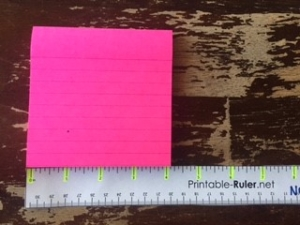When the location moved to begin at 4 inches, the actual length of the post-it did not change, it is still 4 inches long, though the location now ends at 8 inches.To print paper rulers visit https://printable-ruler.net/wp-content/uploads/2015/08/ruler-12in-30cm-USLetter-metallic.pdf.

For more information on Measuring Length with Fractions, visit Learn Zillion at https://learnzillion.com/resources/64165-using-fractions-in-measurement-data.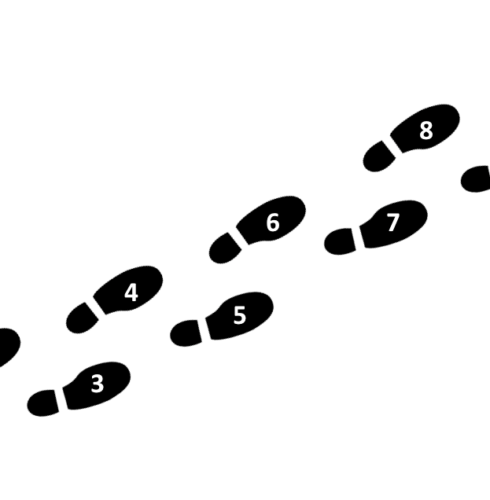According to the standard, MAFS.K.CC.1.1, students are required to, “Count to 100 by ones and by tens.” During the school day, there are many opportunities for students to practice rote counting such as:

Counting the number of chairs in the classroom
Counting a set of objects
Counting the number of students in the class
Counting through songs
Counting sets of 10 fingers or full ten frames

The practice of rote counting helps to reinforce the counting sequence. It is important to scaffold the count sequence as students begin to rote count. For example, students might begin rote counting from 0-10, as they show fluency, the sequence can be extended. Students might also practice rote counting during transition times as they count the steps they take (as seen in the image below).After students develop an understanding of rote counting, MAFS.K.CC.1.2, requires students to, “Count forward beginning from a given number within the known sequence.” An application of this standard is to use a set of cards (0-99) to practice counting forward from a given number. Person A selects a card and Person B has to name the next number in the counting sequence (see image below).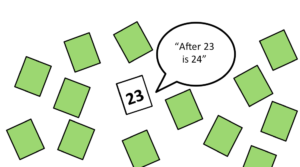This task should be differentiated based on rote counting ability. Students should be able to rote count 0-20 before they are asked to count forward from 6, etc.

For more ideas check out this link: http://www.cpalms.org/Public/PreviewResourceStudentTutorial/Preview/107684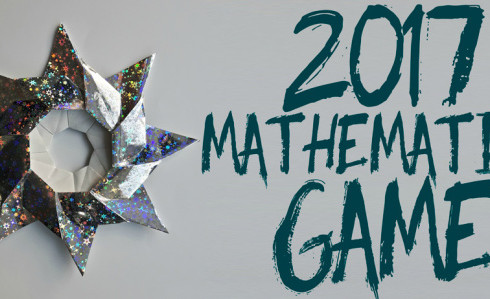It’s January, and the beginning of a new calendar year. The NCTM Math Forum annually holds a contest for students to determine if they can use the digits in the year along with any of the operation symbols, a decimal point, and/or exponents to write expressions for each of the values 0-100.

Which ones are possible? Are any impossible with this year’s digits? The NCTM Math Forum has printable worksheets and manipulatives for children to use to solve and record the possible expressions.Checkout this video from www.teachingchannel.org to see students build fact fluency as they discover how 1-digit multiplication can help with 2- and 3-digits, too. This is a great example of students engaging in “SMP 7: Look for and make use of Structure”, and “SMP 8: Look for and express regularity in repeated reasoning.”Students in 3rd grade are expected to measure length to the nearest whole inch, half inch and quarter inch. (See the item specifications listed below).It is helpful to begin this learning by relating one whole inch to one whole on a number line. To do this, magnify one whole inch on a larger piece of paper to have students break the inch into fractional amounts by folding and relating to fractions on a number line. Follow the steps below:

1. Pass out 1 sentence strip to each student.

2. Have the students fold the sentence strip into fourths and cut.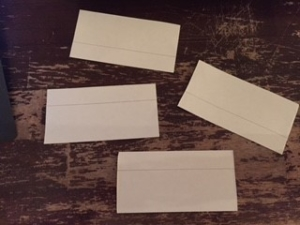3. Then represent one of those pieces of paper as 1 whole inch “magnified.”4. Take one smaller piece of paper. Label the 0 and 1 on the paper, then fold down the middle. Pose the question, “What does this middle line represent?” Discuss how it is half of a whole inch so that mark represents ½ inch.5. Take another small piece of paper and fold down the middle, then fold in half again. Point to the first mark after 0 and pose the question, “What does this mark represent?” Have students explore and discuss that it represents 1 out of 4 spaces traveled on the paper so it’s ¼ of an inch. Continue this process with 2/4, ¾ and 1.6. Compare and contrast how we determined fractions of an inch to fractions on a number line in previous units. Pose a question such as, “How do the fractions of an inch compare to fractions of a whole?”7. Use the last piece of paper to explore a different range, such as 2-3 and fold to determine where 2 ½ would be located and other fractions within that range. Depending on how your students seem to be doing, may want to continue with other ranges to 12.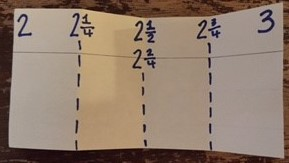8. Compare and contrast magnified inches to rulers – Pass out rulers to have students look for the fractions of a whole inch. Have them compare and contrast their paper magnified models to the actual ruler.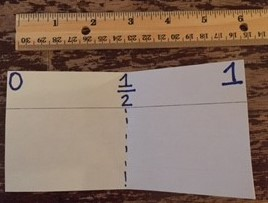For more information on developing understanding of fractions within a whole inch, visit the CPALMS Magnified Inch Lesson at http://www.cpalms.org/Public/PreviewResourceLesson/Preview/46871.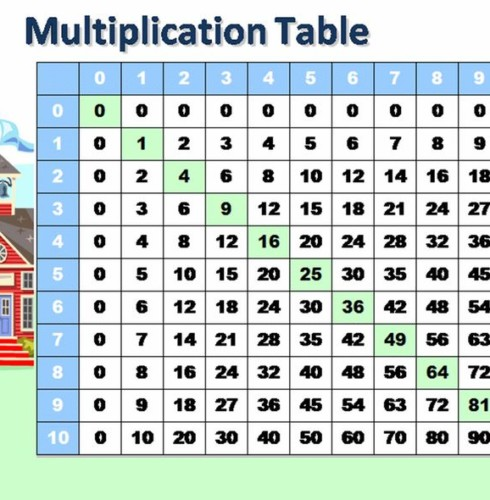While the 3rd grade standard MAFS.3.OA.3.7 states, “By the end of grade 3, know from memory all products of two one-digit numbers.”, this is, unfortunately, not always the case. There are often 4th grade students who are attempting to build an understanding of strategies for multi-digit multiplication while still lacking basic fact fluency. While fluency with basic facts is not essential for understanding strategies such as partial products, it sure helps.

MAFS.3.OA.3.7 also states that students should, “Fluently multiply and divide within 100, using strategies such as the relationship between multiplication and division…or the properties of operations.” That doesn’t exactly sound like the rote memorization of facts that we were all subjected to. So, what are those strategies they used in 3rd grade?Below are several videos that explore a variety of strategies which support students as they build multiplication and division fluency.

This first video provides a brief explanation of the benefits of teaching students strategies versus wrote memorization of the multiplication table:
“>

The following brief videos go into more detail regarding some specific strategies, and may provide you with some insight into how to support those students who are still working towards fluency:
“>
“>
“>
“>
“>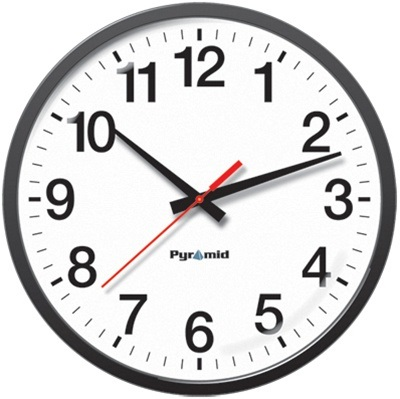In 3rd grade, students are expected to:
• Tell and write time to the nearest minute
• Use analog and digital clocks to tell time
• Measure time intervals in minutes
• Solve word problems that involve addition and subtraction of time intervals in minutes and represent the problems on a number line.

Telling time is an important concept for everyday life. It’s also one that students some time struggle with if they don’t understand how a clock works. One reason students struggle with telling time on analog clock is the world around us has digital clocks. Another reason is because students focus on telling time during the measurement unit, and then move to a different concept.

One way to help students tell time is repetition with quick tasks throughout the year.
An activity that could help with that is:

It’s That Time!
• If you have a digital clock up in your classroom – Place pictures of analog clock times on index cards or small pieces of paper and pass out to a few students each day. When it is the time on the given analog clock, the child would stand up and announce “It’s that time” to the rest of the students. Everyone would compare the card to the actual clock to see if they agree, if not problem solve to determine the misconception.• If you have an analog clock up in your classroom – Place pictures of digital clock times on index cards or small pieces of paper and pass out to a few students each day. When it is the time on the given digital clock, the child would stand up and announce “It’s that time” to the rest of the students. Everyone would compare the card to the actual clock to see if they agree, if not problem solve to determine the misconception.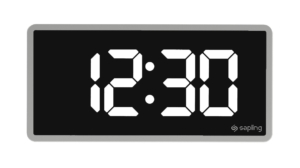Extension – have students record the analog and digital time on a blank clock page.

• Encourage your school to place a schoolwide analog clock on the classroom televisions.
• As a parent, purchase an analog wrist watch for the child. This will encourage them to understand how the clock works with repetition.
• Record the class schedule using analog clocks on the board.
• Put a time matching game as a center where students can match the digital and the analog clocks with the same time.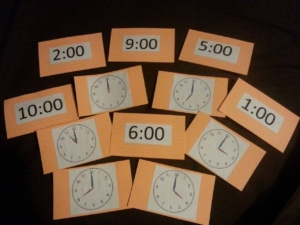• Give time riddles. For example, “The hour hand is halfway between the 3 and 4, what time is it?” We would want students to justify it’s 3:30 because it’s halfway between the hour of 3 and 4.
• Encourage students to continue practicing telling time on an analog clock at home. For spiral homework, you could have them record three events that happen throughout a night and record the digital and analog time for each event.

For more ideas on telling time on an analog clock, read the related article at http://maccss.ncdpi.wikispaces.net/file/view/Taking+Time+to+Understand+Time.pdf.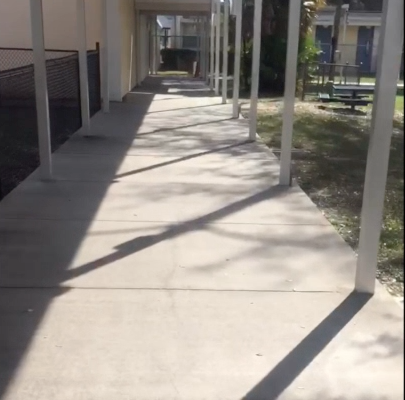We’ve all heard the saying, “The shortest distance between two points is a straight line.”

Last week, as I was walking around a school, I thought to myself, is that saying true? Are there certain routes within a school building that take less time to travel than others? What would be the benefits of taking the faster route?

I videotaped myself walking to the same location taking two different routes.
Which route is the most efficient route to walk?
Do they both take the same amount of time?
How might you determine which route the is best route to travel?

Check out these videos below!!

Option A

Option B

Happy Problem Solving!!!Looking for a way to refine your math lessons? It is tough to go this alone, so why not work together with your peers. Lesson Studies are a fantastic approach in working together as a team to share, develop, and refine great ideas in teaching math.

A great place to begin is by focusing on selecting an excellent math goal and task. This link can get you started and provide direction in implementing a lesson study with a team or other group of like-minded math teachers at your school.In 3rd grade, students are expected to generate simple equivalent fractions.

Example: 1/2 = 4/8 and 4/6 = 2/3.

Models should be used to help students identify equivalent fractions. Sentence strips or strips of paper cut into congruent rectangles can be used to model the idea of equivalency.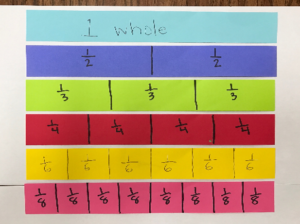Students will build conceptual understanding of equivalent fractions through the use of area and linear models. Number lines should be often used to model as it is an efficient strategy for finding equivalent fractions.As the standard states, students should be able to “explain why fractions are equivalent e.g, by using a visual fractional model”. The use of procedures or algorithms is not a 3rd grade expectation. Students will have more success and understanding when they can prove if two fractions are equal or not.

Learn Zillion – Recognize Equivalent Fractions Using Number Lines: https://learnzillion.com/lesson_plans/4739-recognize-equivalent-fractions-using-number-lines

Comparing using a number line –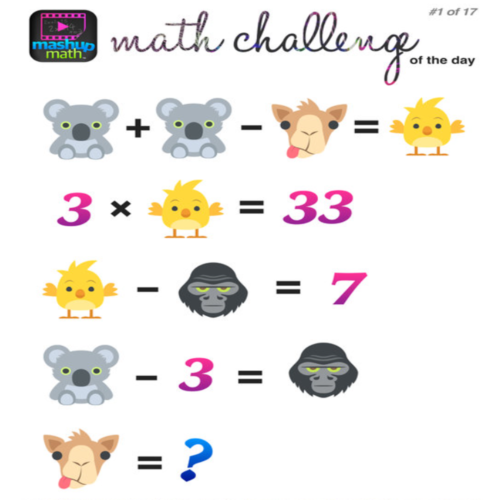Check out the website MashUp Math for awesome math challenges! These are great way to get students excited about math and stretch their algebraic thinking!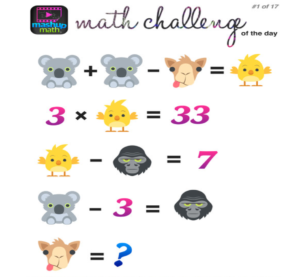In 3rd grade, students are expected to compare two fractions that have either the same numerator or the same denominator. When comparing fractions, it is important for students to understand the value of the fraction and consider both the numerator and denominator and their relationship to each other. So be cautious of teaching statements such as:

“The smaller the denominator, the larger the fraction.”

“The larger the denominator, the smaller the fraction.”

It is important for students to begin comparing fractions with a concrete representation, such as the area model to help them understand the size of the parts and number of parts.Using representations to compare fractions help students develop their number sense about fraction size. This awareness helps them to understand the strategies they use to compare whole numbers do not necessarily compare to fractions (1/2 is greater than 1/6 even though the whole number 6 is greater than 2). Through hands on experience, students will understand that the larger the digit in the denominator the smaller the piece.Make sure these symbols are used when comparing fractions:

< less than > greater than

= equal toThese Learn Zillion videos support students understanding of comparing fractions with the same denominator or numerator:

Compare fractions to the same whole: https://learnzillion.com/lesson_plans/7801-compare-fractions-to-the-same-whole

Compare fractions with the same numerator: https://learnzillion.com/lesson_plans/8217-compare-fractions-with-the-same-numerator-using-the-greater-than-and-less-than-symbols